## 硬派越野哈弗H9 你身邊的“超級英雄”

2018年11月28日 11:55 來源：車行天下 超過：9723次關注

越野的世界總是令人向往的。里面充滿著冒險與挑戰，他們離開平坦的城市柏油路，離開紅綠燈，離開那些呆板的交通指示牌和規矩的斑馬線。他們挑戰山川、征服高原荒野、穿越沙漠、攀爬虎克之路。他們的世界和膽識、謀略、力量、勇氣有關，這是每個男人都希望的生活。工欲善其事必先利其器，想要越野，首選你得購置一臺硬派越野車，是越野車而不是SUV。而市面上現行的硬派越野幾乎都在50萬-300萬的價格區間內，這其中有日系代表豐田酷路澤，英系代表路虎衛士，美系代表Jeep牧馬人，德系代表奔馳G65。所以說，越野有時候并不僅僅是膽識與勇氣的比拼，其中還需要金錢這個硬性指標。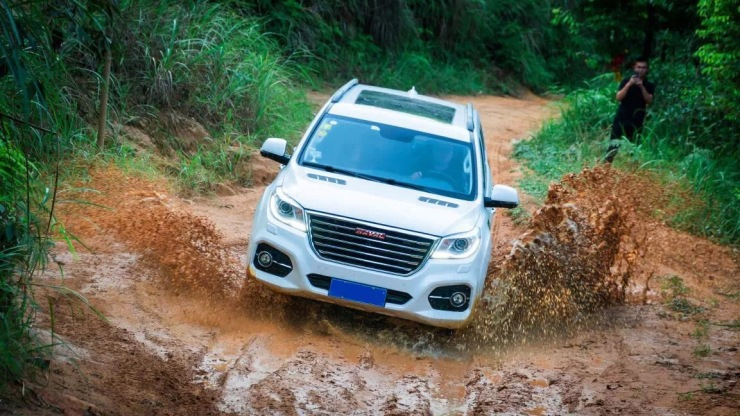當然，凡事既有例外，隨著國內車企技術研發實力的成熟，也出現了不少價格實惠，性能優異的硬派越野車，而這其中的佼佼者，便是哈弗旗下的旗艦車型—哈弗H9，它也是中大型SUV市場中唯一的國產硬漢。作為國產硬漢，哈弗H9-8AT搭載了2.0T+8AT的動力總成，其中2.0T雙流道汽油發動機，最大功率185kW，最大扭矩355N·m;2.0T雙渦輪柴油發動機最大功率140kW，最大扭矩420N·m;擁有非承載式車身，前雙叉臂獨立懸架、后多連桿非獨立懸架，雙速TOD智能四驅后橋電子差速鎖等等硬派越野車專屬構造。此外，哈弗H9的接近角達到28°，離去角達到23°，最小離地間隙更是達到206mm，優異的通過性，讓它可以在戶外盡情“撒歡”。非常值得一提的還有哈弗H9的全地形控制系統，擁有AUTO、運動、沙地、雪地和泥地等七個模式，其中4L模式能將低扭放大2.48倍，可輕松攀爬60%坡度。駕駛者只需通過中控臺的旋鈕便能輕松切換七種模式，簡單快捷，即便是越野初獵者也能很快上手。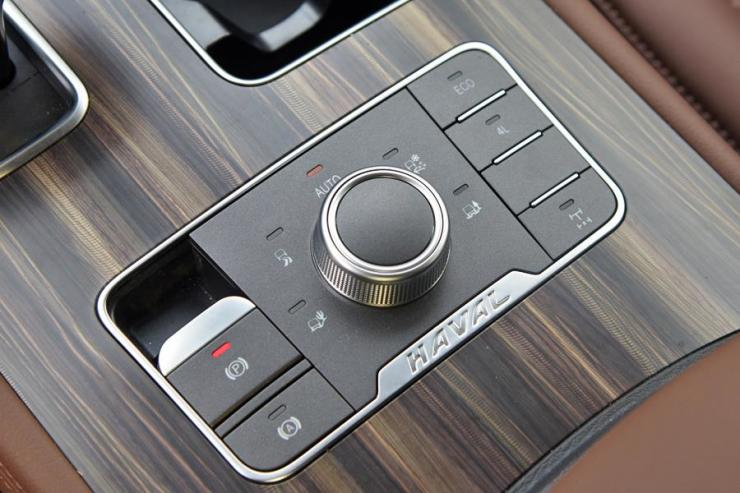也正是這些越野利器，奠定了哈弗H9在自主越野中的領導者地位，今年1-10月，哈弗H9銷量達12570輛，累計同比增長23.15%，在中大型SUV市場銷量放緩的背景下，顯得很是亮眼。哈弗H9能夠取得如此優異的市場成績，與哈弗H9出色的越野性能，可靠的質量品質以及極高的性價比密切相關。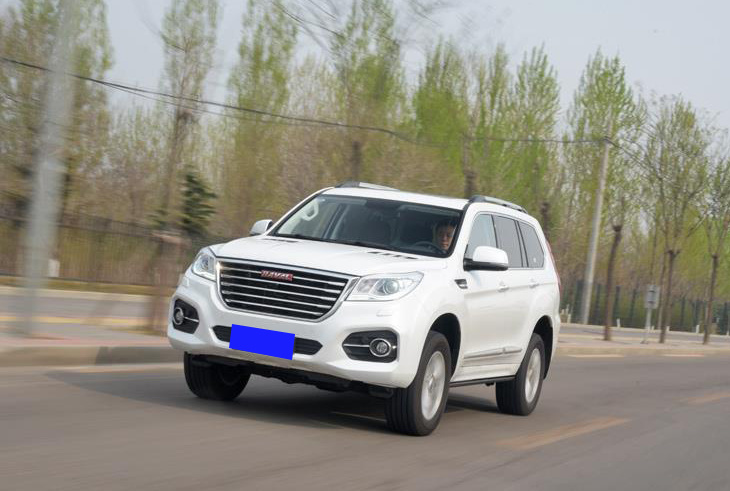當然，更離不開消費者對于這款中大型SUV市場上唯一的國產硬漢，發自內心的喜愛。對于消費者來說，哈弗H9已經不單單只是一款汽車產品，它更像是國產硬派越野高度的象征。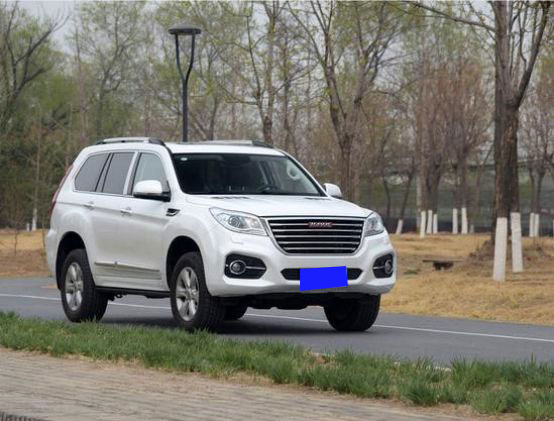這種說法并不是空穴來風，也不是平地拔高。舉個例子，在國防領域，曾經國防用車幾乎都是豐田陸巡、三菱帕杰羅等車型，而隨著自主品牌的興起與技術的越發成熟，如今，哈弗H9現已被選為國防用車，已經在云南、內蒙古、廣西、新疆、天津等其他15個省、自治區及直轄市服役，未來還將繼續擴大份額。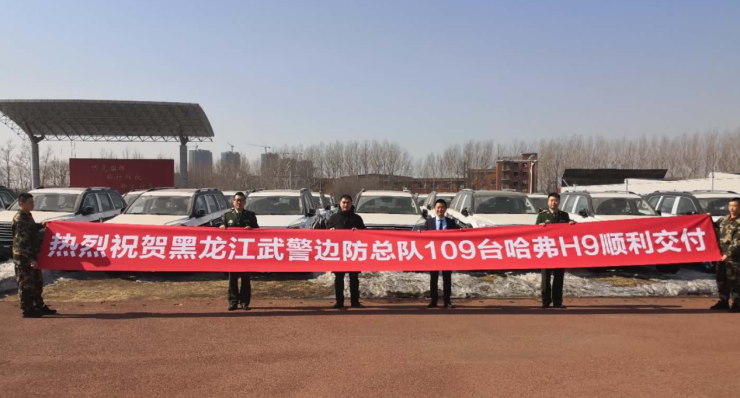當然，國防用車不是兒戲，質量安全與越野性能直接關系到戰士的生命安全，而哈弗H9被選入國防用車，也側面證明了其產品質量與越野性能。事實上，不僅國內，哈弗H9在國外也備受歡迎。日前，柬埔寨和孟加拉國軍警系統也都向哈弗采購了大批量哈弗H9作為其軍方指定用車。

而在前段時間的俄羅斯車展上，哈弗H9也受到大家的追捧，擁有霸氣的外觀和全面的機能的哈弗H9對于有著“戰斗民族”的俄羅斯民眾來說可是巨大的誘惑。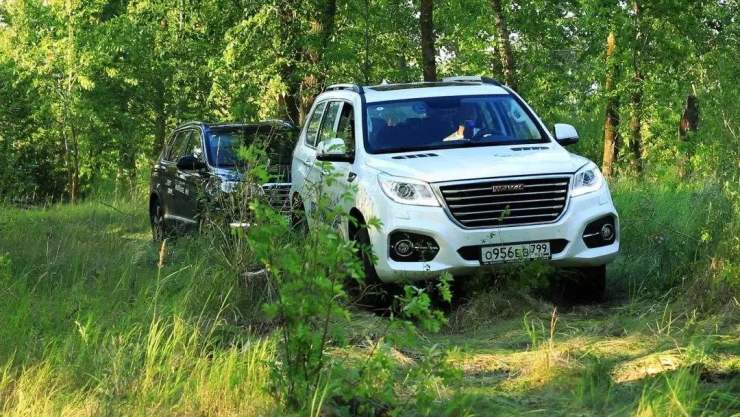不僅于此，哈弗H9更是國內自主品牌首款打破“20萬天花板”的車型，在哈弗H9上市以前，自主品牌難有售價突破20萬的產品，這部分市場被合資品牌牢牢把控，即便偶爾出現一兩輛自主車型，也如曇花一現，消失無蹤。而哈弗H9自2014年上市以來，不僅在售價上率先擊穿自主20萬元的天花板，與合資車型同臺競技，且銷量持續上升，獲得了眾多消費者的認可。可以說，哈弗H9在某種程度上為后來的自主企業樹立了信心，其象征地位不言而喻。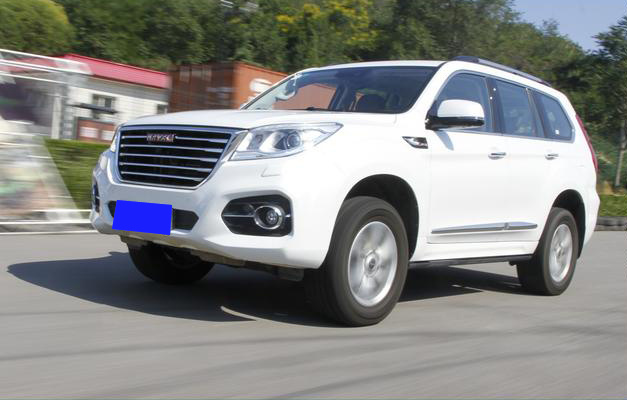寫在最后：

哈弗H9在產品質量，越野性能方面都有非常不錯表現，并且憑借著較為親民的價格，更是成為不少越野愛好者的首選車型。但哈弗H9的存在并不僅局限于此，它更像是哈弗在中國越野車領域樹立的一面旗幟，如同提及日系越野會聯想到陸巡，美系會想到牧馬人一樣，談起中國越野，你肯定會聯想到哈弗H9。

#### 相關文章

0-500 字已有評論 0條 查看評論>>

?
• 快速找車
• 選擇品牌
• 選擇品牌
• A  奧迪
• A  阿斯頓·馬丁
• A  阿爾法·羅密歐
• B  寶沃
• B  布加迪
• B  巴博斯
• B  保時捷
• B  賓利
• B  奔馳
• B  寶馬
• B  本田
• B  別克
• B  標致
• B  比亞迪
• B  寶駿
• B  北汽制造
• B  北汽新能源
• B  北汽幻速
• B  北汽威旺
• B  北京汽車
• B  奔騰
• B  北汽紳寶
• C  長安
• C  長安商用
• C  長城
• C  昌河
• D  大眾
• D  道奇
• D  DS
• D  東南
• D  東風風神
• D  東風風行
• D  東風小康
• D  東風風度
• D  東風
• F  福特
• F  豐田
• F  菲亞特
• F  法拉利
• F  福田
• F  福迪
• F  福汽啟騰
• G  觀致
• G  廣汽傳祺
• G  廣汽吉奧
• G  GMC
• H  紅旗
• H  漢騰汽車
• H  哈弗
• H  哈飛
• H  海格
• H  海馬
• H  華頌
• H  黃海
• H  華泰
• H  恒天
• J  吉利汽車
• J  捷豹
• J  Jeep
• J  江淮
• J  江鈴
• J  金杯
• J  九龍
• J  金旅
• K  凱翼
• K  凱迪拉克
• K  克萊斯勒
• K  科尼塞克
• K  卡威
• K  開瑞
• L  路虎
• L  林肯
• L  勞斯萊斯
• L  蘭博基尼
• L  雷克薩斯
• L  鈴木
• L  雷諾
• L  理念
• L  力帆
• L  蓮花汽車
• L  獵豹
• L  路特斯
• L  陸風
• M  馬自達
• M  MG
• M  MINI
• M  瑪莎拉蒂
• M  摩根
• M  邁凱輪
• N  納智捷
• O  歐寶
• O  謳歌
• O  歐朗
• Q  奇瑞
• Q  起亞
• Q  啟辰
• R  日產
• R  榮威
• R  瑞麒
• S  三菱
• S  斯威汽車
• S  薩博
• S  smart
• S  斯柯達
• S  斯巴魯
• S  思銘
• S  雙龍
• S  上汽大通
• S  雙環
• T  特斯拉
• T  騰勢
• W  沃爾沃
• W  五菱汽車
• W  五十鈴
• W  威茲曼
• W  威麟
• X  現代
• X  雪佛蘭
• X  雪鐵龍
• X  西雅特
• Y  一汽
• Y  英菲尼迪
• Y  英致
• Y  依維柯
• Y  野馬汽車
• Y  永源
• Z  眾泰
• Z  中華
• Z  中興
• Z  知豆
• 選擇車系
• 選擇車系
• 車型對比
• 選擇品牌
• 選擇品牌
• A  奧迪
• A  阿斯頓·馬丁
• A  阿爾法·羅密歐
• B  寶沃
• B  布加迪
• B  巴博斯
• B  保時捷
• B  賓利
• B  奔馳
• B  寶馬
• B  本田
• B  別克
• B  標致
• B  比亞迪
• B  寶駿
• B  北汽制造
• B  北汽新能源
• B  北汽幻速
• B  北汽威旺
• B  北京汽車
• B  奔騰
• B  北汽紳寶
• C  長安
• C  長安商用
• C  長城
• C  昌河
• D  大眾
• D  道奇
• D  DS
• D  東南
• D  東風風神
• D  東風風行
• D  東風小康
• D  東風風度
• D  東風
• F  福特
• F  豐田
• F  菲亞特
• F  法拉利
• F  福田
• F  福迪
• F  福汽啟騰
• G  觀致
• G  廣汽傳祺
• G  廣汽吉奧
• G  GMC
• H  紅旗
• H  漢騰汽車
• H  哈弗
• H  哈飛
• H  海格
• H  海馬
• H  華頌
• H  黃海
• H  華泰
• H  恒天
• J  吉利汽車
• J  捷豹
• J  Jeep
• J  江淮
• J  江鈴
• J  金杯
• J  九龍
• J  金旅
• K  凱翼
• K  凱迪拉克
• K  克萊斯勒
• K  科尼塞克
• K  卡威
• K  開瑞
• L  路虎
• L  林肯
• L  勞斯萊斯
• L  蘭博基尼
• L  雷克薩斯
• L  鈴木
• L  雷諾
• L  理念
• L  力帆
• L  蓮花汽車
• L  獵豹
• L  路特斯
• L  陸風
• M  馬自達
• M  MG
• M  MINI
• M  瑪莎拉蒂
• M  摩根
• M  邁凱輪
• N  納智捷
• O  歐寶
• O  謳歌
• O  歐朗
• Q  奇瑞
• Q  起亞
• Q  啟辰
• R  日產
• R  榮威
• R  瑞麒
• S  三菱
• S  斯威汽車
• S  薩博
• S  smart
• S  斯柯達
• S  斯巴魯
• S  思銘
• S  雙龍
• S  上汽大通
• S  雙環
• T  特斯拉
• T  騰勢
• W  沃爾沃
• W  五菱汽車
• W  五十鈴
• W  威茲曼
• W  威麟
• X  現代
• X  雪佛蘭
• X  雪鐵龍
• X  西雅特
• Y  一汽
• Y  英菲尼迪
• Y  英致
• Y  依維柯
• Y  野馬汽車
• Y  永源
• Z  眾泰
• Z  中華
• Z  中興
• Z  知豆
• 選擇車系
• 選擇車系
• 選擇車型
• 選擇車型
• 意見反饋